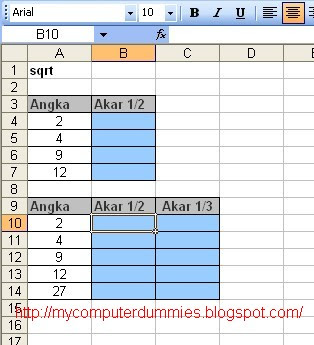## Tuesday, 28 July 2009

### Function square root (square)

Microsoft excel provides a function to calculate (calculate) the root of a number, you can calculate the square root, cube root, and so on.

Here is a simple example:

1. Create a table like the following
(table with a light blue background, not fill because the content will be filled a formula)2. In cell B4 content of the formula:

= SQRT (A4)

other than the above formula you can use the formula:
a. B10 cells, you can calculate the square root of the number of cells with the formula A10:

= A10 ^ 0.5

b. or in cell C 10, you can calculate the cube root of the number of cells with the formula A10:

= A10 ^ (1 / 3)

the result will look like the image below